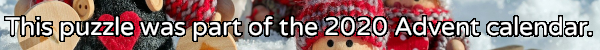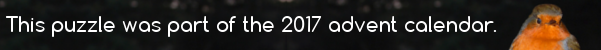mscroggs.co.uk
mscroggs.co.uksubscribe

# Puzzles

## 21 DecemberThere are 3 ways to order the numbers 1 to 3 so that no number immediately follows the number one less that itself:
• 3, 2, 1
• 1, 3, 2
• 2, 1, 3
Today's number is the number of ways to order the numbers 1 to 6 so that no number immediately follows the number one less that itself.

## 13 DecemberThere are 6 ways to split the sequence of the numbers 1 to 5 into three shorter sequences:
• 1 and 2 and 3, 4, 5
• 1 and 2, 3 and 4, 5
• 1 and 2, 3, 4 and 5
• 1, 2 and 3 and 4, 5
• 1, 2 and 3, 4 and 5
• 1, 2, 3 and 4 and 5
Today's number is the number of ways to split the sequence of the numbers 1 to 10 into five shorter sequences.

## 18 DecemberToday's number is the maximum number of pieces that a (circular) pancake can be cut into with 17 straight cuts.

## 15 DecemberIf the numbers 1 to 7 are arranged 7,1,2,6,3,4,5 then each number is either larger than or a factor of the number before it.
How many ways can the numbers 1 to 7 be arranged to that each number is either larger than or a factor of the number before it?

## Archive

Show me a random puzzle
▼ show ▼